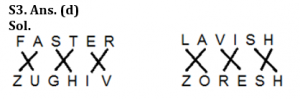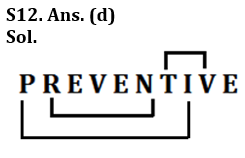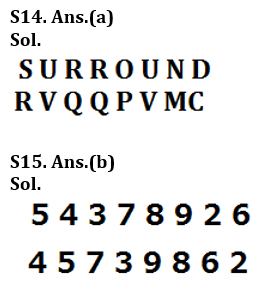Latest Banking jobs   »

# Reasoning Ability Quiz For IDBI AM/ Bank of India PO 2023 -12th March

Q1. How many meaningful words of four letters can be formed using first two and the last two letters of the word ‘LONGEST using each letter only once’?
(a) One
(b) Two
(c) Three
(d) Four
(e) None

Q2. How many such pairs of letters are there in the word ‘CLEANEST’, each of which has as many letters between them in the word (in both forward and backward directions) as they have between them in the English alphabetical series?
(a) One
(b) Two
(c) Three
(d) More than three
(e) None

Q3. In a certain code language, ‘FASTER’ is coded as ‘ZUGHIV’ and ‘SUMMER’ is coded as ‘FHNNIV’, then ‘LAVISH’ will be coded as ‘—‘.
(a) ORSHEZ
(b) MTKPOV
(c) KPTMVO
(d) ZORESH
(e) None of these

Directions (4): Study the given information carefully and answer the given questions.
Twenty persons are standing in a straight line, facing north. There are only three students between J and K. L is standing exactly between K and J. There are more than four students between J and M. M is standing sixth to the right of L. N is standing fourth from the right end of the line. J is standing sixth from the left end.

Q4. How many people are standing between N and M?
(a) One
(b) Two
(c) Three
(d) None
(e) More than three

Q5. Which of the following will come in place of the question mark (?) in the following series based on the English alphabetical series?
BDA FHE JLI ?
(a) OPM
(b) NPM
(c) PMN
(d) PMO
(e) None of these

Directions (6-10): These questions are based on the following six numbers.

412 646 734 255 536 876

Q6. If we add 2 to the second digit of each number and subtract 1 from the first digit of each number, then which of the given will become the lowest number?
(a) 646
(b) 536
(c) 255
(d) 412
(e) None of these

Q7. If all the digits in each of the numbers are written in increasing order within than number, than which of the given number will become the second highest?
(a) 412
(b) 646
(c) 255
(d) 536
(e) None of these

Q8. If the first two digits of each number are interchanged, then which of the following numbers will become the highest number?
(a) 734
(b) 536
(c) 646
(d) 412
(e) 876

Q9. In each number, if we subtracted 1 in first digit and added 1 in last digit, then which among these number will be 2nd lowest number?
(a) 734
(b) 255
(c) 876
(d) 412
(e) None of these

Q10.If we replace all the odd digits in each number with zero, then which of the following number will become the lowest?
(a) 734
(b) 536
(c) 412
(d) 255
(e) 876

Q11. In a certain code language ‘DOME’ is written as ‘8943’, and ‘MEAL’ is written as ‘4321’. What group of letters can be formed for the code ‘38249’?
(b) MEDOA
(c) EMDAO
(d) EDAMO
(e) MEAOD

Q12. How many such pairs of letters are there in the word “PREVENTIVE”, each of which has as many letters between them in the word as in the English alphabet?
(a) None
(b) One
(c) Two
(d) Three
(e) More than three

Q13. If it is possible to make only one meaningful word with the first, third, fifth and the ninth letters of the word ‘CURVATURE’, which would be the second letter of the word? If more than one such word can be formed, give X as the answer. If no such word can be formed, give K as your answer.
(a) R
(b) C
(c) X
(d) E
(e) K

Q14. Each vowel in the word SURROUND is changed to the next letter in the English alphabet and each consonant is changed to the previous letter in the English alphabet. Which of the following will be the second from the right end in the new arrangement?
(a) M
(b) C
(c) V
(d) P
(e) R

Q15. The positions of the first and the second digits of the number 54378926 are interchanged. Similarly, the positions of the third and the fourth digits are interchanged and so on. Which of the following will be the sixth digit from the left end after the rearrangement?
(a) 6
(b) 8
(c) 9
(d) 2
(e) 7

Solutions

S1. Ans. (c)
Sol. LOTS, SLOT, LOST

S2. Ans. (b)S4. Ans. (b)

S5. Ans. (b)

Solution (6-10):
S6. Ans. (c)
S7. Ans. (b)
S8. Ans. (e)
S9. Ans. (d)
S10. Ans. (a)

S11. Ans. (d)
Sol. If DOME=8943, MEAL=4321, 38249=EDAMOS13. Ans. (c)
Sol. CURVATURE. The letter is = C, R, A, E
We can form two words from above letter= RACE, CARE, ACRE## FAQs

### What is the selection process of the IDBI AM?

The selection process of IDBI AM is Online exam and Interview.

#### Congratulations!Union Budget 2023-24: Free PDF